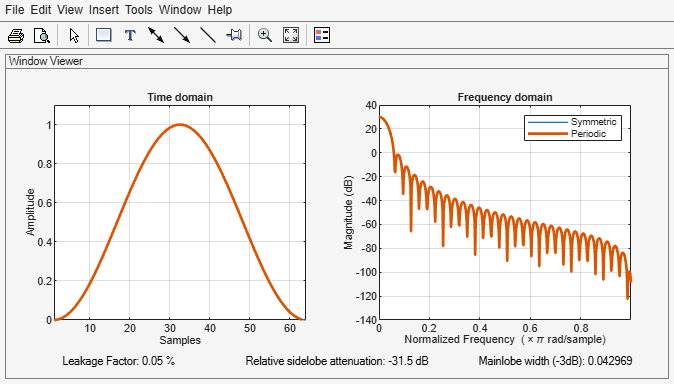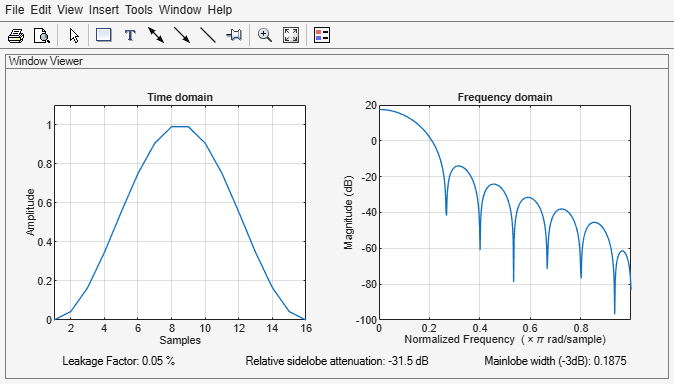# winwrite

Class: sigwin.hann
Package: sigwin

Save Hann window object values in ASCII file

## Syntax

```winwrite(H) winwrite(H,'filename') ```

## Description

`winwrite(H)` opens a dialog to export the values of the Hann window object `H` to an ASCII file. The file extension `.wf` is automatically appended.

`winwrite(H,'filename')` saves the values of the Hann window object `H` in the current folder as a column vector in the ASCII file `'filename'`. The file extension `.wf` is automatically appended to `filename`.

## Examples

expand all

Generate two Hann windows:

• The first window has N = 64 and is symmetric.

• The second window has N = 63 and is periodic.

Display the two windows.

```Hs = sigwin.hann(64,'symmetric'); Hp = sigwin.hann(63,'periodic')```
```Hp = Name: 'Hann' SamplingFlag: 'periodic' Length: 63 ```
```wvt = wvtool(Hs,Hp); legend(wvt.CurrentAxes,'Symmetric','Periodic')```Generate a symmetric Hann window with N = 16. Return the window values as a column vector. Show information about the window object. Display the window.

```H = sigwin.hann(16); win = generate(H)```
```win = 16×1 0 0.0432 0.1654 0.3455 0.5523 0.7500 0.9045 0.9891 0.9891 0.9045 ⋮ ```
`wininfo = info(H)`
```wininfo = 4x26 char array 'Hann Window ' '----------- ' 'Length : 16 ' 'Sampling Flag : symmetric' ```
`wvtool(H)`